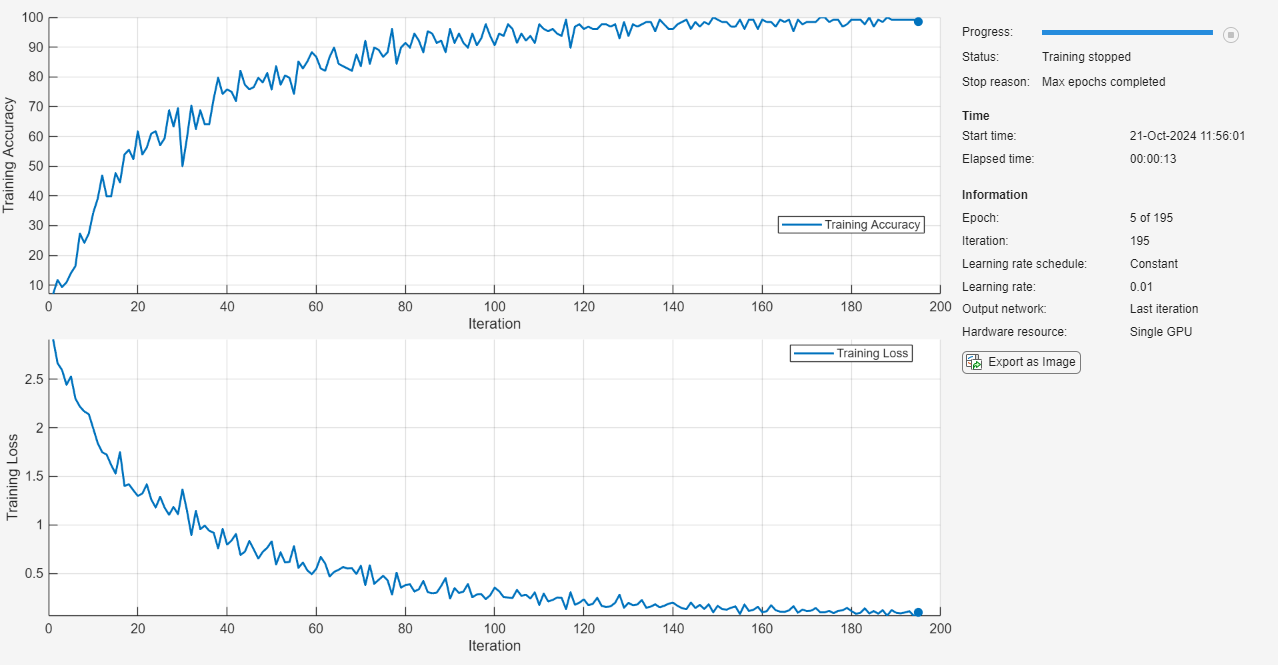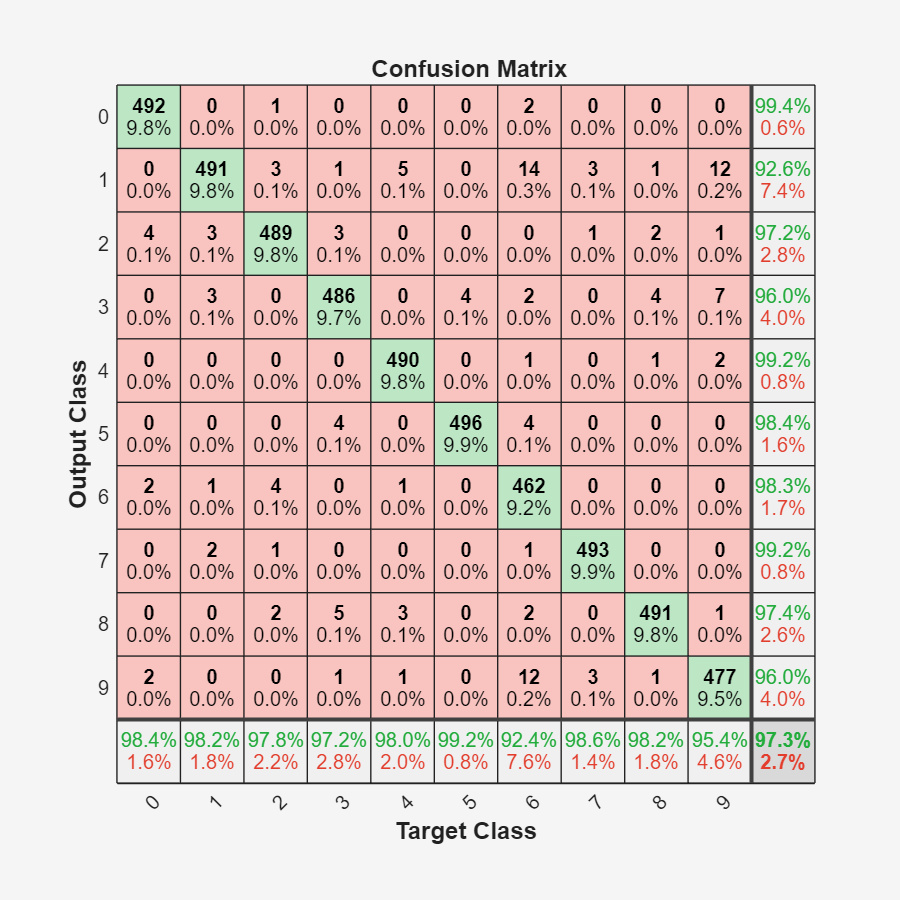# plotconfusion

Plot classification confusion matrix

## Syntax

``plotconfusion(targets,outputs)``
``plotconfusion(targets,outputs,name)``
``plotconfusion(targets1,outputs1,name1,targets2,outputs2,name2,...,targetsn,outputsn,namen)``

## Description

example

````plotconfusion(targets,outputs)` plots a confusion matrix for the true labels `targets` and predicted labels `outputs`. Specify the labels as categorical vectors, or in one-of-N (one-hot) form.On the confusion matrix plot, the rows correspond to the predicted class (Output Class) and the columns correspond to the true class (Target Class). The diagonal cells correspond to observations that are correctly classified. The off-diagonal cells correspond to incorrectly classified observations. Both the number of observations and the percentage of the total number of observations are shown in each cell.The column on the far right of the plot shows the percentages of all the examples predicted to belong to each class that are correctly and incorrectly classified. These metrics are often called the precision (or positive predictive value) and false discovery rate, respectively. The row at the bottom of the plot shows the percentages of all the examples belonging to each class that are correctly and incorrectly classified. These metrics are often called the recall (or true positive rate) and false negative rate, respectively. The cell in the bottom right of the plot shows the overall accuracy.```
````plotconfusion(targets,outputs,name)` plots a confusion matrix and adds `name` to the beginning of the plot title.```
````plotconfusion(targets1,outputs1,name1,targets2,outputs2,name2,...,targetsn,outputsn,namen)` plots multiple confusion matrices in one figure and adds the `name` arguments to the beginnings of the titles of the corresponding plots.```

## Examples

collapse all

Load the data consisting of synthetic images of handwritten digits. `XTrain` is a 28-by-28-by-1-by-5000 array of images and `YTrain` is a categorical vector containing the image labels.

```[XTrain,YTrain] = digitTrain4DArrayData; whos YTrain```
``` Name Size Bytes Class Attributes YTrain 5000x1 6062 categorical ```

Define the architecture of a convolutional neural network.

```layers = [ imageInputLayer([28 28 1]) convolution2dLayer(3,8,'Padding','same') batchNormalizationLayer reluLayer convolution2dLayer(3,16,'Padding','same','Stride',2) batchNormalizationLayer reluLayer convolution2dLayer(3,32,'Padding','same','Stride',2) batchNormalizationLayer reluLayer fullyConnectedLayer(10) softmaxLayer classificationLayer]; ```

Specify training options and train the network.

```options = trainingOptions('sgdm',... 'MaxEpochs',5,... 'Verbose',false,... 'Plots','training-progress'); net = trainNetwork(XTrain,YTrain,layers,options);```Load and classify test data using the trained network.

```[XTest,YTest] = digitTest4DArrayData; YPredicted = classify(net,XTest);```

Plot the confusion matrix of the true test labels `YTest` and the predicted labels `YPredicted`.

`plotconfusion(YTest,YPredicted)`The rows correspond to the predicted class (Output Class) and the columns correspond to the true class (Target Class). The diagonal cells correspond to observations that are correctly classified. The off-diagonal cells correspond to incorrectly classified observations. Both the number of observations and the percentage of the total number of observations are shown in each cell.

The column on the far right of the plot shows the percentages of all the examples predicted to belong to each class that are correctly and incorrectly classified. These metrics are often called the precision (or positive predictive value) and false discovery rate, respectively. The row at the bottom of the plot shows the percentages of all the examples belonging to each class that are correctly and incorrectly classified. These metrics are often called the recall (or true positive rate) and false negative rate, respectively. The cell in the bottom right of the plot shows the overall accuracy.

Load sample data using the `cancer_dataset` function. `XTrain` is a 9-by-699 matrix defining nine attributes of 699 biopsies. `YTrain` is a 2-by-699 matrix where each column indicates the correct category of the corresponding observation. Each column of `YTrain` has one element that equals one in either the first or second row, corresponding to the cancer being benign or malignant, respectively. For more information on this dataset, type `help cancer_dataset` at the command line.

```rng default [XTrain,YTrain] = cancer_dataset; YTrain(:,1:10)```
```ans = 2×10 1 1 1 0 1 1 0 0 0 1 0 0 0 1 0 0 1 1 1 0 ```

Create a pattern recognition network and train it using the sample data.

```net = patternnet(10); net = train(net,XTrain,YTrain);```

Estimate the cancer status using the trained network. Each column of the matrix `YPredicted` contains the predicted probabilities of each observation belonging to class 1 and class 2, respectively.

```YPredicted = net(XTrain); YPredicted(:,1:10)```
```ans = 2×10 0.9999 0.9999 0.9999 0.0578 0.9993 0.9999 0.0012 0.0001 0.0028 0.9999 0.0001 0.0001 0.0001 0.9422 0.0007 0.0001 0.9988 0.9999 0.9972 0.0001 ```

Plot the confusion matrix. To create the plot, `plotconfusion` labels each observation according to the highest class probability.

`plotconfusion(YTrain,YPredicted)`In this figure, the first two diagonal cells show the number and percentage of correct classifications by the trained network. For example, 446 biopsies are correctly classified as benign. This corresponds to 63.8% of all 699 biopsies. Similarly, 236 cases are correctly classified as malignant. This corresponds to 33.8% of all biopsies.

5 of the malignant biopsies are incorrectly classified as benign and this corresponds to 0.7% of all 699 biopsies in the data. Similarly, 12 of the benign biopsies are incorrectly classified as malignant and this corresponds to 1.7% of all data.

Out of 451 benign predictions, 98.9% are correct and 1.1% are wrong. Out of 248 malignant predictions, 95.2% are correct and 4.8% are wrong. Out of 458 benign cases, 97.4% are correctly predicted as benign and 2.6% are predicted as malignant. Out of 241 malignant cases, 97.9% are correctly classified as malignant and 2.1% are classified as benign.

Overall, 97.6% of the predictions are correct and 2.4% are wrong.

## Input Arguments

collapse all

True class labels, specified one of the following:

• A categorical vector, where each element is the class label of one observation. The `outputs` and `targets` arguments must have the same number of elements. If the categorical vectors define underlying classes, then `plotconfusion` displays all the underlying classes, even if there are no observations of some of the underlying classes. If the arguments are ordinal categorical vectors, then they must both define the same underlying categories, in the same order.

• An N-by-M matrix, where N is the number of classes and M is the number of observations. Each column of the matrix must be in one-of-N (one-hot) form, where a single element equal to 1 indicates the true label and all other elements equal 0.

Predicted class labels, specified one of the following:

• A categorical vector, where each element is the class label of one observation. The `outputs` and `targets` arguments must have the same number of elements. If the categorical vectors define underlying classes, then `plotconfusion` displays all the underlying classes, even if there are no observations of some of the underlying classes. If the arguments are ordinal categorical vectors, then they must both define the same underlying categories, in the same order.

• An N-by-M matrix, where N is the number of classes and M is the number of observations. Each column of the matrix can be in one-of-N (one-hot) form, where a single element equal to 1 indicates the predicted label, or in the form of probabilities that sum to one.

Name of the confusion matrix, specified as a character array. `plotconfusion` adds the specified `name` to the beginning of the plot title.

Data Types: `char`